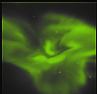Next: Evaluation Up: Geometrical calibration of ALIS Previous: System and requirements   Contents

# Calibration methods

For studies of the atmosphere and the ionosphere it is appealing to use calibration images made under the same conditions with exactly the same rotations and use background stars as a way of determining the lines-of-sight. In this way the refraction of light in the atmosphere is automatically accounted for.

The requirement that the determination of the viewing directions should be accurate to within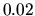implies that the sky positions of these stars need to be known to within. This accuracy requirement demands that the sky positions of the stars have to be rigorously corrected for precession when calculated from the star catalogue. The star catalogue used in this work is the Yale Bright Star catalogue (YBS) which contains selected stars down to a magnitude 6. In order to verify the accuracy of the position calculations, the results have been compared with the star chart program xephem (available at the time of writing in version 3.2.3). It is not necessary to correct for the nutation, the minor variation in the inclination of the earth axis due to the torque from the moon. Likewise it is not necessary to include the corrections for aberration, proper motion and parallax in the calculations. These higher order corrections are smaller than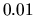.

To proceed with determining the pixel lines-of-sight it is necessary to be able to identify a large number of stars in an image, preferably more than 100 evenly distributed over the image plate. For these star pixels'' we know the lines-of-sight to within the required accuracy. For the intermediate pixels we can choose either to interpolate between the lines-of-sight from neighbouring star pixels or use an optical transfer function that describes where the light from a direction in spacefalls on the image. The identified stars are used to adjust the parameters of the optical transfer function so that the calculated directions of the stars are mapped down to their respective points on the image.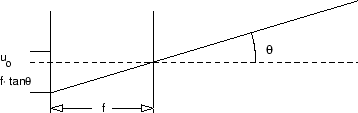For practical work the first approximation of an optical transfer function is the pinhole camera model, which is described in most textbooks on optical imaging ( Gonzalez and Woods, 1993); see Figure 4.2. The incident light from a direction in space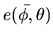is projected onto image coordinates according to: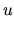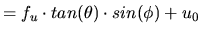(4.4)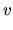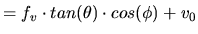(4.5)

whereare the horizontal and vertical coordinates in the image,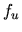andare the horizontal and vertical focal widths,and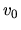are the horizontal and vertical projection of the optical axis, and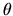andare polar angle relative to the optical axis and the azimuthal angle around the optical axis. The first attempt to determine the lines-of-sight of all pixels in the image data from the ALIS cameras with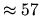fields-of-view made use of the pinhole camera model, but with unsatisfactory results. With careful identification of a large number of stars in several images, it was found that a significant systematic error remained after optimisation, as can be seen in Figure 4.3 for which the radial error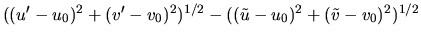is plotted against the radial position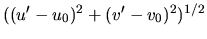. Here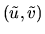are the image coordinates of the calculated star position and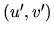are the identified image positions of the stars.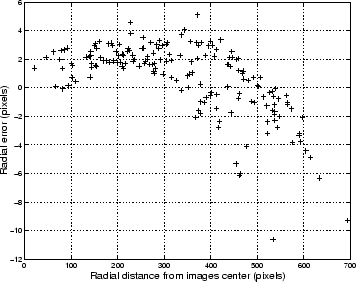In Figure 4.3 it can clearly be seen that for small radial distances the pinhole camera maps light too close to the centre of the image and for larger angles too far from it. In order to compensate for the error in the radial direction it is sufficient to include a term that is linear in the polar angle relative to the optical axis. This leads to a modified optical transfer function: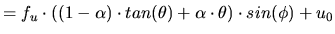(4.6)(4.7)

After a new fit of the optical parameters for the modified optical model, it appears that the systematic error has been removed (see Figure 4.4).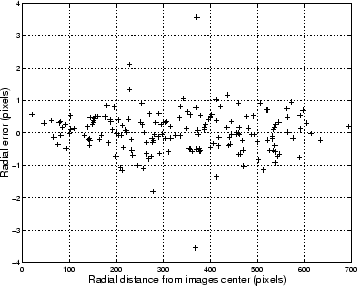For the auroral and atmospheric studies which are the focus of ALIS, where the aim is to determine the position of objects at altitudes between 15 and 500 km, the required parameter is the path of the light through the atmosphere.

Due to the variations in density and temperature with altitude, the refractive index of the atmosphere varies from 1 in free space to approximately 1.0003 at sea level. This variation in refractive index causes an object outside the atmosphere to appear closer to zenith than it actually is. An everyday example of this is that when the sun disappears from the horizon its centre is already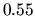below the horizon.

For a flat plane parallel atmosphere the total change in zenith angle is easily calculated by Snell's law: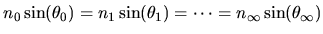(4.8)

whereis the refractive index and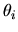is the zenith angle at altitude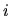. From this it is straightforward to calculate the difference between the true zenith angle,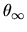, and the apparent zenith angle,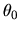, where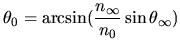(4.9)

This simple relation is correct to withinfor zenith angles up to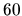; for larger zenith angles it is not possible to neglect the curvature of the earth. A more general correction that is good for zenith angles up tois ( Smart, 1977):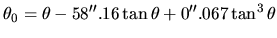(4.10)

In order to determine the path through the atmosphere there are three choices: to use the apparent zenith angle and to perform a path tracing in order to calculate the true path of the light, to use the apparent zenith angle and assume that the light travels in a straight line, or to use the true zenith angle and use that angle for calculating the line-of-sight.

If we use the true zenith as the zenith angle of the light ray there will be an error between that ray and the true path of light due to the refraction in the atmosphere as can be seen in Figure 4.5,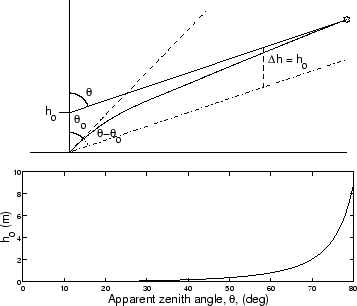for which the ray paths are plotted from the ground to the sky. The error will be equivalent to an increase in the altitude of the observing site. This displacement error in the altitude of the observation site,, can be expressed as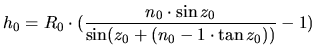(4.11)

For zenith angles of up to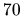this accounts for a negligible error of less thanm, ( Green, 1985).

If we were to use the apparent zenith angle, the error between the infered and the true paths of light would be: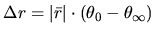(4.12)

For a zenith angle of 45the difference between true and apparent zeniths is approximately 1 arc minute which gives an error of approximately 60 m at 200 km distance. Here it can be concluded that use of the true zenith angles and approximations of the light path with a straight line gives errors that are within the acceptable accuracy. The small error that results from this approximation can be compared with all other errors in the analysis and weighted against the computationally heavy and cumbersome task of performing a path-tracing through an atmosphere whose refractive index structure as a function of altitude is, at best, only roughly known.Next: Evaluation Up: Geometrical calibration of ALIS Previous: System and requirements   Contents

copyright Björn Gustavsson 2000-10-24